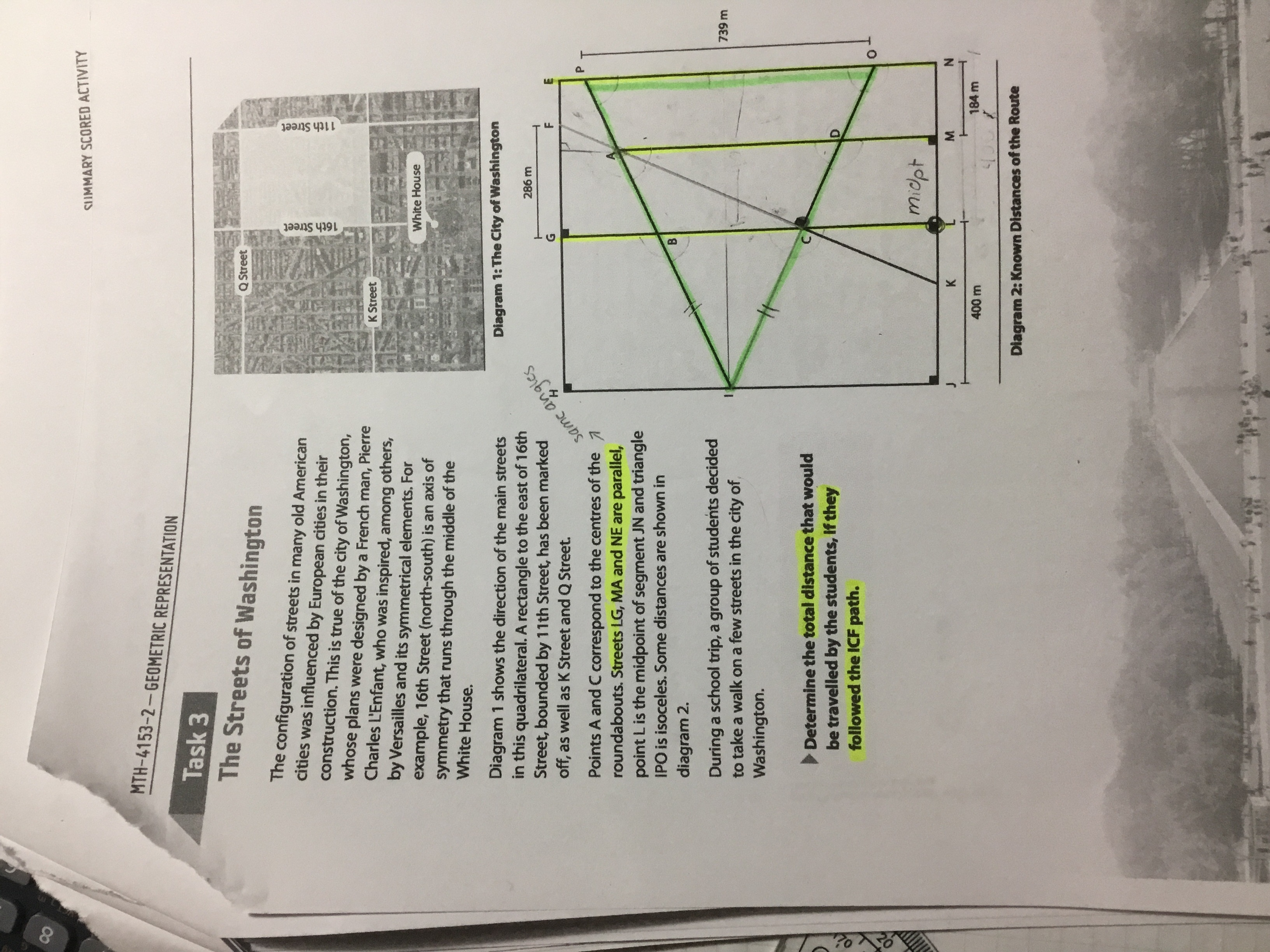# Help Zone

### Student Question

Hi, I’ve been stuck on a maths problem. It’s pretty big…You can explain in french too if you like I know this isn’t an english platform. It’s on secondary 4 CST trigonometry.Mathematics## Explanations (1)

•Explanation from AlloprofExplanation from Alloprof

This Explanation was submitted by a member of the Alloprof team.

Hello Crocodile!

Thank you for using our platform :)

First of all, you need to find the length of IC and then CF.

To find the length of the IC segment, you will need several key concepts. As you have well indicated, the segments IB and IC are equal, since it is an isosceles triangle.

In order to find the length of IC, you can use trigonometry once you have the segment BC and the height of the triangle IBC.

You already have the height, but you do not know the length of the base. To find it, you could use the dimensions of similar triangles IBC and IOP. We know they are similar because the lines are parallel. Thus, they have the same angles. You can find the k factor with the height of the triangles which is shown in the picture. Now you just need to apply it to the base of the IOP triangle to find the base of the IBC triangle.

After, since it is an isosceles triangle, you can divide this value by two and use pythagora's theorem to find the segment IC.

Hope this gives you a good starting point. I encourage you to complete the number. Do not hesitate if you have other questions, it will be a real pleasure for me to answer you :)

Elizabeth :D